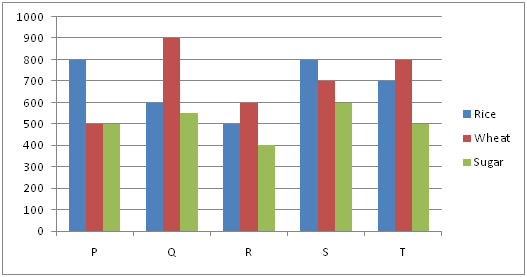Today I'm going to discuss a problem related to bar charts in Data interpretation. In almost every bank exam, one set is always asked from this section in Quantitative aptitude. I'm going discuss the problem with a same pattern which has already been asked in so many exams.

## Steps to solve problems of Data Interpretation: Bar-Charts

• Study the chart first and have an idea about the values presented in the form of bar-charts.
• To make the problem simple get an idea about the range of values presented in bar-chart.
• If you want to save your time, read the question and just concentrate on bar-chart itself and do the calculations on it.
Problem1: Study the following graph and answer the questions:X-axis is represented as different restaurants i.e. P, Q, R, S and T and Y- axis is represented as consumption of rice, wheat and sugar in kilograms.

1. Find the ratio of amount of rice, wheat and sugar consumed by restaurant Q to the amount consumed by restaurant T.

2. Find the average amount of rice, wheat and sugar consumed by all restaurants.

3. Rice consumed by restaurant R is what percent of rice consumed by all restaurants?

4. What is the difference between the average amount of wheat and average amount of sugar consumed by all restaurants together?

Solution: First of all, study the chart and analyze it. You will come to know the following points:

• X-Axis to Restaurants (P, Q, R, Sand T)
• Y-Axis to Amounts in kilograms (Lies between 400-900)
• Three bars to Rice, Wheat and Sugar
Now, lets start solving the questions:

1. Amount of rice, wheat and sugar consumed by restaurant Q  = 600+ 900+ 550
⇒2050
Amount consumed by restaurant T = 700+ 800+ 500
⇒ 2000

Now, Ratio of both of above value i.e. Q: T
⇒ 2050 = 2000
⇒ 41: 40

2. To find average amount of rice, wheat and sugar consumed by all restaurants.
Amount of rice, wheat and sugar consumed by restaurant:

P = 800+ 500+ 500= 1800
Q = 600+ 900+ 550= 2050
R = 500+ 600+ 400= 1500
S = 800+ 700+ 600= 2100
T = 700+ 800+ 500= 2000

Average = (1800+ 2050+ 1500+ 2100+ 2000)/5

⇒(9450)/5
⇒1890

3. Let Rice consumed by restaurant R = x% of rice consumed by all restaurants together
Let  Rice consumed by restaurant R = A i.e. 500
rice consumed by all restaurants together= B i.e. 800+ 600+ 500+ 800+ 700 = 3400

So, A = x% of B

⇒ x= A/B times 100

⇒ x= (500)/(3400) times 100

⇒x = 14.7%

4. Difference between the average amount of wheat and average amount of sugar consumed by all restaurants together.

Average amount of wheat by all restaurants = A =(500+ 900+ 600+ 700+ 800)/5

⇒3500/5

⇒ 700

Average amount of sugar by all restaurants = B =(500+ 550+ 400+ 600+ 500)/5

⇒2550/5

⇒510

Difference = A-B = 700- 510

⇒ 190kg

You are free to ask anything. I will try to update more problems soon.
Problem related to Data interpretation: Tables.

Method to solve problems related to Bar-Charts.Reviewed by Jasleen Behl on Sunday, January 19, 2014 Rating: 5